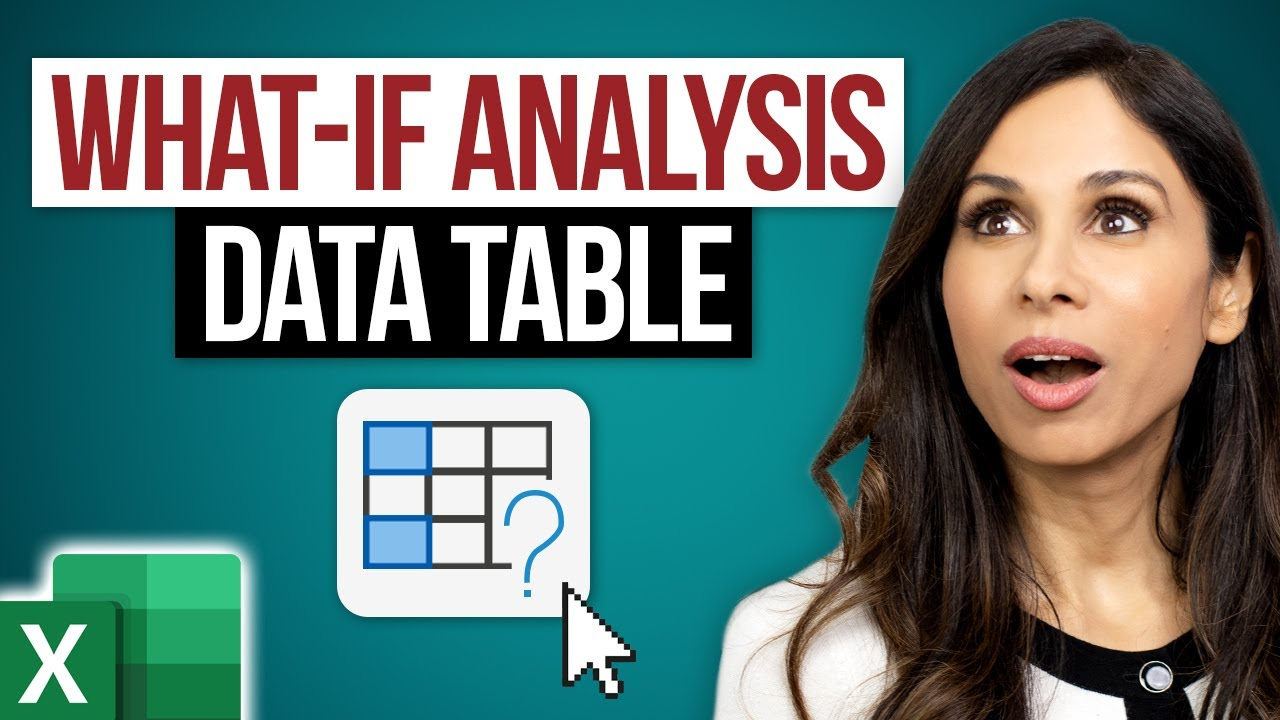en | deDec 2, 2022 12:00 AM

# Excel What-If Analysis Data Table – Easy to Use Once you Learn This

## Use an Excel Data Table What-if Analysis to quickly create multiple results based on a formula you already have.

Use an Excel Data Table What-if Analysis to quickly create multiple results based on a formula you already have. The data table in Excel will allow you to change values in some cells and come up with different answers to the problem. This is what Microsoft Excel's What-if Analysis Data Table does best - it allows you to experiment with different input variables. A Data Table allows you to see how different input values would impact the result at one glance. It's quite a hidden Excel tip and trick. Data Tables can be confusing at first, but this tutorial explains it in simple English and in a way, you will never forget how to use this feature again.

In this example we’ll create a retirement savings plan. You'll calculate how much money you will have after saving a specific amount of money per month over a number of years. Our inputs for the calculation are the monthly payments, the number of years we're paying into the retirement fund, and the interest rate earned. We’ll use a Data Table in Excel to explore what happens to the future value of the fund if the interest rate or years changes. Data tables recalculate every time you make changes in the workbook. There is a setting you can tweak to stop data tables from calculating. Go to Formulas / Calculation Options / Automatic Except Data Tables. You can toggle this option on or off depending on your needs.

## In this Video:

00:00 What-If Analysis in Excel - Most Simple Explanation

00:16 Data Table - 1 Variable

04:47 Bonus Trick: Custom Formatting as icon

05:55 Data Table - 2 Variables

08:07 Wrap Up

HubSite 365 Apps